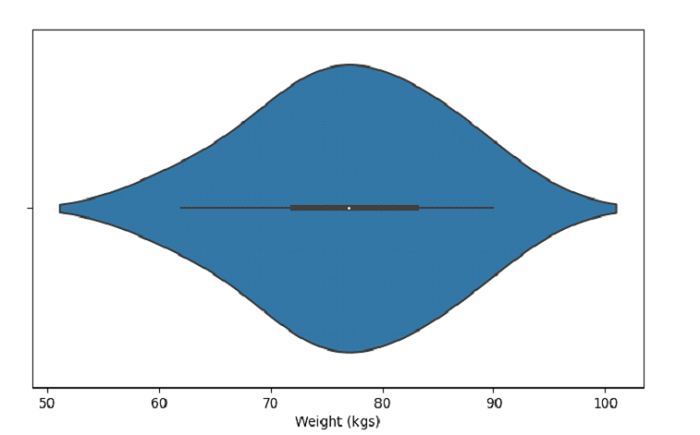# Python Pandas - Draw a single horizontal violinplot with Seaborn

Violin Plot in Seaborn is used to draw a combination of boxplot and kernel density estimate. The seaborn.violinplot() is used for this. Plot a sinle violinplot usinga single column.

Let’s say the following is our dataset in the form of a CSV file − Cricketers.csv

At first, import the required libraries −

import seaborn as sb
import pandas as pd
import matplotlib.pyplot as plt

Load data from a CSV file into a Pandas DataFrame −

dataFrame = pd.read_csv("C:\Users\amit_\Desktop\Cricketers.csv")


Plotting single horizontal violin plot with Weight (kgs) column −

sb.violinplot(dataFrame['Weight'])

## Example

Following is the code −

import seaborn as sb
import pandas as pd
import matplotlib.pyplot as plt

# Load data from a CSV file into a Pandas DataFrame

# plotting single horizontal violin plot with Weight (kgs) column
sb.violinplot(dataFrame['Weight'])

# set label
plt.xlabel("Weight (kgs)")

# display
plt.show()

## Output

This will produce the following output −Updated on: 04-Oct-2021

148 Views